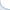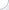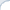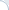Getting up to speed with neural machine translation : How not to burn yourself with PyTorch

Last week I shared my time between work and hacking at a forth machine translation marathon in the Americas. This event organized jointly by CMU and Amazon (sponsored by Amazon) was a lot of fun. My small sub-team of two people got familiar with OpenNMT, trained English-German and English-Ukrainian models, as well as implemented an idea of our team lead Adithya Renduchintala. Hey, we have even gotten a tiny 0.5 gain in BLEU for the English-Ukrainian pair!

We certainly learned a lot of lessons, most of which generalize well beyond the neural machine translation task. One is related to implementation of custom neural modules and PyTorch. Unlike TensorFlow and many other packages, PyTorch belongs to a new crop of neural frameworks, where a neural network (computation) graph is dynamic. What does it mean? It means that you do not have define a computation graph in advance. You can simply write a tensor-manipulating code and PyTorch will do all the back-propagation and parameter updating automatically. Another well-known package with a similar functionality is DyNet.

There are ups and downs to dynamic computation graphs. For one thing, it is much simpler to debug them. For another, there is a lot of magic going behind the scenes, which you need to understand. First of all, one needs to remember that the computation graph is defined by a sequence of manipulations on Tensors and Variables (Variable is a Tensor wrapper that got deprecated in the recent PyTorch). Your sequences should be valid and properly linked so that all the Tensors of interest have a chance to be updated during back-propagation.

Tensor-level manipulations can easily get hairy. To simplify things, PyTorch introduces an abstraction layer called Module. A Module is a basic building block that has some parameters and a function forward to turn inputs to outputs. If all is done properly, given only the forward function PyTorch can compute the gradients via back-propagation and update the model parameters. The nice thing about PyTorch is that you can easily write a new module by combining several existing ones. There is no need to write arcane description of layers! As we can see from this PyTorch example, we can define a forward network computation in a very straightforward way. Even if you have not seen a line of PyTorch code before, you can easily figure out that this module applies two 2d-convolutions each of which is followed by a RELU non-linearity:

1. class Model(nn.Module):
2. def __init__(self):
3. super(Model, self).__init__()
4. self.conv1 = nn.Conv2d(1, 20, 5)
5. self.conv2 = nn.Conv2d(20, 20, 5)
6.
7. def forward(self, x):
8. x = F.relu(self.conv1(x))
9. return F.relu(self.conv2(x))

But here is a catch that is barely mentioned in PyTorch documentation. Although writing the forward function is sufficient to compute the gradients, it is apparently not sufficient to determine which tensors represent module's parameters. In the above example, the module includes two convolutional neural networks, each of which has parameters to be updated. How does PyTorch know this? Well, turns out that PyTorch overloads the function __setattr__! Thus, it surreptitiously "registers" each submodule when a user makes an assignment like this one:

1. self.conv1 = nn.Conv2d(1, 20, 5)

Unfortunately, such automatic registration does not work all the time. Imagine, for example, you want to aggregate several sub-modules whose number is not known in advance. It is very natural to save them all in a list:

1. class Model(nn.Module):
2. def __init__(self):
3. super(Model, self).__init__()
4. self.sub_modules = []
5. self.sub_modules.append(nn.Conv2d(1, 20, 5)) # append a module to the list
6. self.sub_modules.append(nn.Conv2d(20, 20, 5)) # append a module to the list

Yet, this is where PyTorch magic stops: If you place the modules in plain Python list, PyTorch will not be able to update their parameters. As a fix, you need to register them explicitly. One way to do this is to explicitly call the function add_module. A less tedious ways is to use a combination of nn.ModuleList and nn.Sequential. Please, read a discussion here for more details.

On adversarial examples and space-partitioning capabilities of neural networks

A tree-based machine learning method splits the space into regions and fits a region-specific classification/regression function (typically just a constant). Additional flexibility and capacity can be achieved by bagging and boosting elementary trees, but at the core of these methods is their space partitioning capabilities. A few months ago I started to wonder if neural networks can do a similar thing. My gut feeling was that neural nets should be doing something of the kind, but the mechanics of the neural net space partitioning was unclear to me. I even posted a question on StackExchange, yet, I have not gotten an answer.

However, I recently discovered an insightful video lecture by Ian Goodfellow where he elaborates on the nature of adversarial examples and discusses possible ways to defend from an attacker (spoiler alert: there seems to be no working method yet!). Basically, he argues that adversarial examples is a common Achilles' heel, which comes—in fact somewhat surprisingly—from excessive linearity of existing approaches. Ian Goodfellow argues that this issue plagues almost all standard machine learning approaches including SVMs, tree-based methods, and neural networks. What is even more surprising, adversarial examples are transferable across different algorithms: An adversarial example designed to fool, e.g., a decision tree is very likely to fool some other method as well!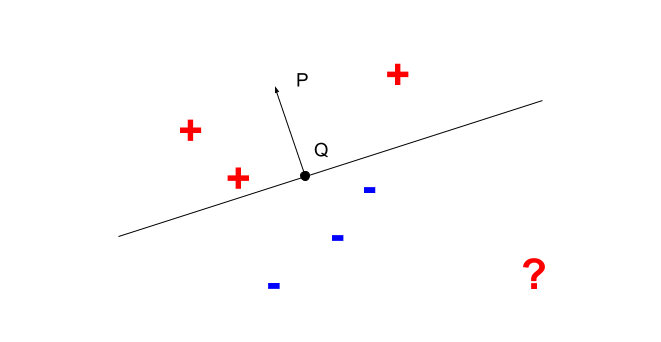Fig 1. A hyperplane separating positive and negative examples.

Ian also notes that neural nets divide the space using an approximately piecewise linear function. This observation basically answers my question about the space partitioning capabilities of neural networks. For neural nets with only truly piecewise linear activation functions (such as RELU), the division of the space is truly linear. As a side comment, this kinda explains (at least for me) the ability of neural networks to generalize quite well despite having so many parameters. A well-known deficiency of the linear and piecewise linear models (as noted by Ian) is that they can be super confident in regions where they encountered no training data. For example, in Figure 1 the linear classifier would have a very strong "opinion" about the negativity of the point marked by the red question mark. However, this confidence is not fully substantiated, because there are no training points nearby.

Adversarial examples notwithstanding, I think it may be useful to get a better understanding of the space partitioning "mechanics" of the neural networks. In my case, such understanding came from reading a very recent paper AN UPPER-BOUND ON THE REQUIRED SIZE OF A NEURAL NETWORK CLASSIFIER by Hossein Valavi and Peter J. Ramadge. The paper is unfortunately paywalled, but the slides are publicly available.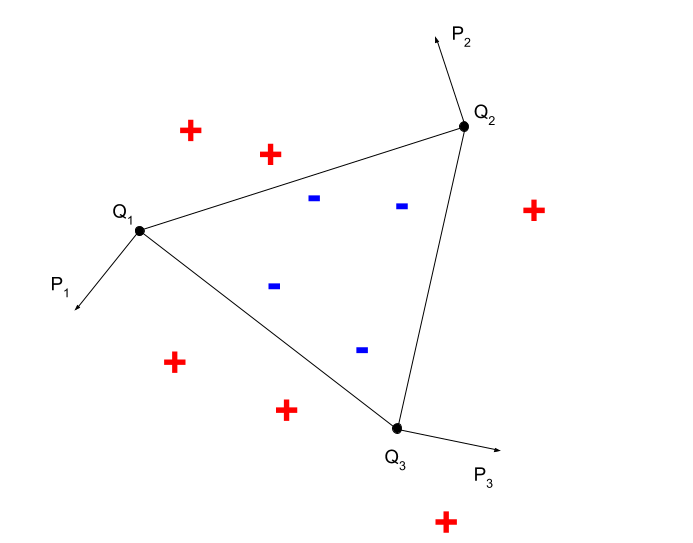Fig 2. A convex shape (triangle) classifier.

In this paper, authors remind us that neural nets possess two powerful capabilities: an ability to distinguish data points separated by a given hyperplane and a capacity to implement arbitrary logic circuits (they also prove facts about complexity of such circuits, but these proofs are not very relevant to our discussion). Without going into technicalities right away (but some are nevertheless discussed below), this means that neural nets can easily divide a real-vector space using polytopes, e.g., convex ones. Let us consider a simple example of a piecewise linear classifier in Fig. 2. As we can see positive and negative training examples can be separated from each other using three planar hyperplanes, simply speaking by three lines. Positive and negative examples are located on the opposite sides of these separating lines. Therefore, a condition of belonging to a negative class can be written as : $\langle x - Q_1, P_1 - Q_1 \rangle < 0 \mbox{ and } \langle x - Q_2, P_2 - Q_2 \rangle < 0 \mbox{ and } \langle x - Q_3, P_3 - Q_3 \rangle < 0$. This condition can be easily implemented with a two layer neural networks with the sign activation function.

Because neural nets can have tons of parameters, they can slice and dice the space at least as well as tree-based learning algorithms. In fact, if necessary, given enough parameters, we can always achieve a perfect separation of data points from different classes (assuming consistent labeling). For example, we can place each positive-class datapoint into its own hyperhube and implement neural nets that "fire" only for training data points in these hypercubes. We will then combine these nets using an OR circuit. Of course, such construct is extremely inefficient and does not generalize well, but it IMHO illustrates the basic space-partitioning powers of the neural nets.

In conclusion, I discuss some technical details, which are primarily of the theoretical interest. For simplicity of exposition I consider only the binary classification scenario. First, let us consider again a hyperplane that divides the space into two subspaces. The claim is that we can always construct a neural network that maps points on one side of the hyperplane to zeros (negative) and points on the other side of the hyperplane to ones (positive). This fact is trivial for neural networks with the sign activation function (a sign of the scalar product between data points and the hyperplane-orthogonal vector defines the class of a data point). We illustrate this point in Figure 1. However, it is more work to prove for differentiable activation functions such as RELU (which are actually used in practice nowadays). This is done in Lemma 3.1 of the mentioned paper.

Once we divide the space into regions and implement all neural networks that fire exactly when a data point is in its region, we need to combine these classifiers using a boolean circuit. Consider again an example in Fig. 2 In this case a boolean circuit is a simple three-variable conjunction.

There are certainly many ways to implement a binary logic circuit using a neural network. One of these is briefly outlined Corollary 3.3. This Corollary is a bit hard to parse, but, apparently, authors propose to solve a problem in two steps. First, they suggest to map each combination of $n$ zeros and ones to a single-coordinate data point in a $2^n$-dimensional space. Because circuit values can be true or false, there will be "true" or "false" data points in the target $2^n$-dimensional space. If coordinate values of true and false points are different, these points are linearly separable (e.g., a "true" data point has exactly one dimension with value one and a "false" data point has exactly one dimension with value two). Thus, according to their Lemma 3.1, there would exist a second neural network based function that maps true points to one and false points to zeros.

Authors do not describe how to implement the mapping to the $2^n$ dimensional space, but we can use the following combination of RELU-activated threshold circuits:

$\max(0, \sum 2^i \cdot x_i - k + 1) - 2\max(0, \sum 2^i \cdot x_i - k) + \max(0, \sum 2^i \cdot x_i - k - 1)$.

Given a binary input variables $x_i$ this function is equal to one if and only if the input is the same as the binary representation of $k$.

UPDATE: quite interestingly a recent paper provides a more specific characterization of functions represented by feed-forward neural networks with RELU activations. Namely, this is a subclass of rational functions belonging to the family of tropical rational maps (a tropical map is piecewise linear).

NLP approaches/tools to automatically rewrite sentences

I was asked on Quora about NLP approaches or tools to automatically rewrite sentences. Here is my brief answer (feel free to vote on Quora).

Not really a specialist in sentence re-writing, but I think at least the following approaches can be used:

1) Manual rules. These can be either regexp based or tree-based. Stanford folks have a tool to create such patterns. One interesting option is to implement rules based on POS tags. I, e.g., reimplemented some of the query-rewriting algorithms from Aranea QA system. This is not easy and the quality is sometimes not ideal.

2) Learned from data. One common approach to do this is, perhaps, surprisingly machine translation. This would require a rather large monolingual corpus of paired sentences. One sentence would be source and another would be a target. You can train all sorts of translation models on such a corpus starting from simplistic Model 1 and ending with some phrase-based model (or even context-free grammar based, see the link below).

3) It is also possible to obtain paraphrasing information by pivoting on a foreign language. Here is one link PPDB: The Paraphrase Database. You may want to read papers authored by the guys who created PPDB (you can use machine translation directly for this as explained by Vasily Konovalov)

4) A more recent approach relies on neural sequence-to-sequence (seq2seq) models. One recent paper on this subject is: Neural Paraphrase Generation with Stacked Residual LSTM Networks, Prakash et al., 2016.

How to declare a constant reference in C++ (not really)

As we may remember, in C++ there are two types of constant pointers. The pointer of the first type (the most common one) can be changed, but not the memory it points to:

1. const int * const_mem = ... ;
2. *const_mem = 3; // compile error
The constant pointer of the second type is basically a reference and it cannot be changed, but you can still change respective memory:
1. int * const const_ptr = ... ;
2. *const_ptr = 3; // fine!
3. const_ptr++; // compile error
Of course, you can define a constant pointer to constant memory as well:
1. const int * const const_ptr_mem = ... ;
2. *const_ptr_mem = 3; // compile error!
3. const const_ptr_mem++; // compile error

References, however, are constant by design. You can assign reference a value only once. You cannot change the reference value afterwards! Thus, references are basically constant non-null pointers. Turns out that you can still define a constant reference in C++:

1. int const & const_ref = 3;
Well, why would such non-sense thing be possible? The answer is that it is not. C and C++ have an extremely quirky way of declaring complex types with complicated rules, which are applied basically in a inside-out right-to-left fashion. Thus, in the previous declaration const still applies to int rather than to int&. In other words, the latter declaration is equivalent to:
1. const int & const_mem_ref = 3;
To declare a true constant reference, which is unsurprisingly illegal, you need to place the modifier const between the '&' and the variable name:
1. int & const const_ref = 3;

Bottom line? Hopefully, reading this short note will help one reduce confusion in the future. As usual, simple illustration code is available.

GCC disables isnan and isinf when compiling with -ffast-math flag

This short note is just a reminder that GCC totally ignores functions isinf and isnan when you compile your code with -ffast-math option. The demo code can be found here. One should also be aware that -ffast-math is enabled by a commonly used option -Ofast, but not by -ON option, where N is a number. I also wrote custom checking functions that do not have such problem.

Pages